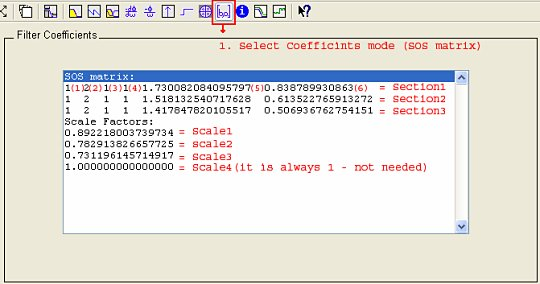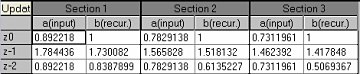# Custom filter import from Matlab

It is possible to import custom filter from Matlab (registered trademark of MathWorks company). Design IIR filter in Matlab and then click on the filter coefficients button. Filter coefficients should appear in SOS matrix form. That is how they are presented in Dewesoft.In Dewesoft you can’t enter the scale factors so you just have to include them in the filter. One section in Dewesoft equals one SOS section in Matlab. All you have to do is scale it the right way. First three coefficients in Matlab are input and are calculated in that way that you multiply the coefficient by the corresponding scale factor. The second three coefficients are recursive and all you need to do is just to copy them from Matlab.

These are calculation formulas for specific section i:

a(input) b(recur.)
z0 Scale i * Section i(1) Section i(4)
z-1 Scale i * Section i(2) Section i(5)
z-2 Scale i * Section i(3) Section i(6)

The coefficients for our example are calculatedbelow: